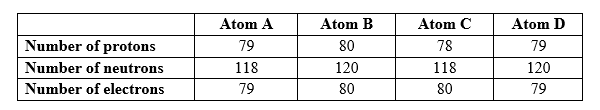# Problem: The table below describes four atoms. Which atoms represent the same element?A) A and B represent the same elementB) A and C represent the same elementC) A and D represent the same elementD) B and C represent the same elementE) C and D represent the same element

###### FREE Expert Solution
90% (138 ratings)
###### Problem Details

The table below describes four atoms. Which atoms represent the same element?

A) A and B represent the same element

B) A and C represent the same element

C) A and D represent the same element

D) B and C represent the same element

E) C and D represent the same elementFrequently Asked Questions

What scientific concept do you need to know in order to solve this problem?

Our tutors have indicated that to solve this problem you will need to apply the Isotopes concept. You can view video lessons to learn Isotopes. Or if you need more Isotopes practice, you can also practice Isotopes practice problems.

What is the difficulty of this problem?

Our tutors rated the difficulty ofThe table below describes four atoms. Which atoms represent ...as low difficulty.

How long does this problem take to solve?

Our expert Chemistry tutor, Dasha took 2 minutes and 41 seconds to solve this problem. You can follow their steps in the video explanation above.

What professor is this problem relevant for?

Based on our data, we think this problem is relevant for Professor Tang's class at USF.